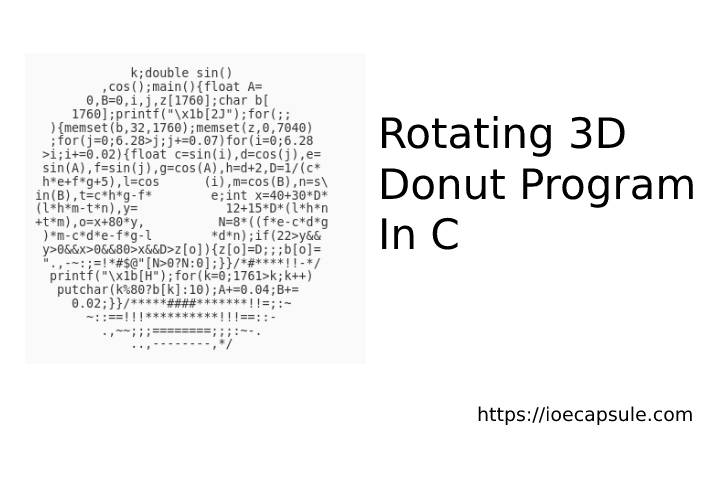### Rotating 3D Donut Program In CC

Recently, I came to know about a program which rotates in 3D creating a nice and smooth graphical view. It uses somewhat complex mathematics and computer graphics terminologies. But if you want just the program, here it is:

``````#include <stdio.h>
#include <math.h>
#include <unistd.h>
#include <string.h>

int main() {
float A = 0, B = 0;
float i, j;
int k;
float z;
char b;
printf("\x1b[2J");
for(;;) {
memset(b,32,1760);
memset(z,0,7040);
for(j=0; j < 6.28; j += 0.07) {
for(i=0; i < 6.28; i += 0.02) {
float c = sin(i);
float d = cos(j);
float e = sin(A);
float f = sin(j);
float g = cos(A);
float h = d + 2;
float D = 1 / (c * h * e + f * g + 5);
float l = cos(i);
float m = cos(B);
float n = sin(B);
float t = c * h * g - f * e;
int x = 40 + 30 * D * (l * h * m - t * n);
int y= 12 + 15 * D * (l * h * n + t * m);
int o = x + 80 * y;
int N = 8 * ((f * e - c * d * g) * m - c * d * e - f * g - l * d * n);
if(22 > y && y > 0 && x > 0 && 80 > x && D > z[o]) {
z[o] = D;
b[o] = ".,-~:;=!*#\$@"[N > 0 ? N : 0];
}
}
}
printf("\x1b[H");
for(k = 0; k < 1761; k++) {
putchar(k % 80 ? b[k] : 10);
A += 0.00004;
B += 0.00002;
}
usleep(30000);
}
return 0;
}
``````

Save the file as `donut.c` and compile it using `gcc donut.c -o donut -lm`

Now, run it using `./donut` . You will see a nice rotating 3d donut on your output display.

If you want to know about how it actually works, please visit the blog post original writer by clicking here -> Donut math: how donut.c works